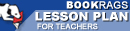Lesson Plans

# Journey Through Genius: The Great Theorems of Mathematics Test | Final Test - Medium

This set of Lesson Plans consists of approximately 142 pages of tests, essay questions, lessons, and other teaching materials.
 View a FREE sampleName: _________________________ Period: ___________________

This test consists of 5 multiple choice questions, 5 short answer questions, and 10 short essay questions.

## Multiple Choice Questions

1. What did Cantor find after extending the continuum between 0 and 1 into two dimensions?
(a) That the set is equal to 1.
(b) That the set is still equal to c.
(c) That the set is infinite.
(d) That the series is infinite.

2. What did George Cantor determine to be true of a set of rational numbers?
(a) They are non-denumerable.
(b) They are all composite numbers.
(c) They are denumerable.
(d) They are all prime numbers.

3. What were the main technique(s) that Euler used to find the sum of the series?
(a) Calculus methods.
(b) Quadratic sums,
(c) Trigonometry and basic algebra.
(d) Cubic equations.

4. Who were Johann and Jakob Bernoulli?
(a) Twin brothers and students of Newton.
(b) Brothers and students of Leibniz.
(c) Cousins and students with Leibniz in Paris.
(d) Cousins and students with Newton at Cambridge.

5. What was described as true about the series 1 + 1/2 + 1/6 + 1/10 + 1/15 + 1/21?
(a) It's a convergent series of cubic numbers.
(b) It's a divergent series with a sum of 2.
(c) It's a convergent series of triangular numbers.
(d) It's a divergent series squared numbers.

## Short Answer Questions

1. What is true about the successive squared denominator series proposed by the Bernoullis?

2. Which of the following is a series that the Bernoullis proposed did not converge on a finite sum?

3. What is the sum of the series 1 + 1/2³ + 1/3³ + 1/4³ ... 1/k³ . . .?

4. What was Dunham central theorem for this chapter?

5. What was most noticeable about Euler at a young age?

## Short Essay Questions

1. Describe who were Jakob and Johann Bernoulli.

2. Describe what mathematical and artistic movements are focused on in the second half of the 19th century.

3. Explain what was the definition of a series before the Bernoullis, and give examples of what was known.

4. Give an example of a series who's sum is still unknown.

5. Describe what the Bernoullis discovered about series, and give an example.

6. Describe Cantor's difficult personal life.

7. Describe the connection between Fermat and Euler's work.

8. Explain any methods used by Cantor that were unsuccessful.

9. What great theorems and work of Newton did Dunham highlight?

10. What was the great theorem of this chapter? Describe it briefly.

(see the answer keys)

 This section contains 916 words (approx. 4 pages at 300 words per page) View a FREE sampleCopyrightsJourney Through Genius: The Great Theorems of Mathematics from BookRags. (c)2019 BookRags, Inc. All rights reserved.
Follow Us on Facebook